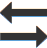# Length converter

 Meter Inch Foot Yard Mile Naut-mile Millimeter Centimeter Decimeter Kilometer League Furlong Rod Mil Angstrom Fathom Pica Point Redshift AU (Astronomical-unit) Light-second Light-minute Light-year ParsecMeter Inch Foot Yard Mile Naut-mile Millimeter Centimeter Decimeter Kilometer League Furlong Rod Mil Angstrom Fathom Pica Point Redshift AU (Astronomical-unit) Light-second Light-minute Light-year Parsec

### Length conversion cheat sheet

#### Metric to U.S

1 centimeter = 0.3937 inch
1 meter = 39.37 inches
1 meter = 1.0936 yards
1 kilometer = 0.6214 mile

#### U.S to Metric

1 inch = 2.54 centimeters
1 foot = 30.48 centimeters
1 foot = 0.3048 meters
1 yard = 0.9144 meters
1 rod = 5.029 meters
1 mile = 1.6093 kilometer

### Units used in this converter

meter, inch, foot, yard, mile, nautical mile, millimeter, centimeter, decimeter, kilometer, league, furlong, rod, mil, Angstrom, fathom, pica, point, redshift, astronomical unit, light second, light minute, light year, parsec